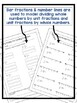# Dividing Fractions Using Models Activity and Word ProblemsSubject
Resource Type
File Type

PDF

(964 KB|18 pages)
Product Rating
4.0
(1 Rating)
Standards
Also included in:
1. Fifth and sixth graders use models to learn how to divide fractions!Included: 4 worksheets increasing in difficulty using a rectangular model for dividing fractions (whole numbers by unit fraction, whole numbers by fractions, fraction by fraction, mixed number by fraction)Answer KeyTeacher Direction
\$5.00
\$4.00
Save \$1.00
• Product Description
• StandardsNEW

Dividing fractions and whole numbers with visual models helps students better understand the why the algorithm works. Models help students gain a deeper understanding of concepts.

Included in this product:

• 7 worksheets using models to divide fractions, which includes whole numbers by unit fractions and unit fractions by whole numbers, solving story problems, writing their own story problems and relating the division problems to multiplication
• Teacher Directions & explanations

This product could be used when you are introducing dividing fractions, as extra practice or small group instruction. It could also be used in centers or as homework.

You may also be interested in a similar, but more difficult Dividing Fractions using Models, a modeling fractions bundle. Or, here's an additional, larger bundle.

Be sure to follow me to be the first to learn about sales and new products!

Be sure to leave feedback to earn TpT credits on future purchases!

Thanks!

Lisa Yeip

Solve real world problems involving division of unit fractions by non-zero whole numbers and division of whole numbers by unit fractions, e.g., by using visual fraction models and equations to represent the problem. For example, how much chocolate will each person get if 3 people share 1/2 lb of chocolate equally? How many 1/3-cup servings are in 2 cups of raisins?
Interpret division of a whole number by a unit fraction, and compute such quotients. For example, create a story context for 4 ÷ (1/5), and use a visual fraction model to show the quotient. Use the relationship between multiplication and division to explain that 4 ÷ (1/5) = 20 because 20 × (1/5) = 4.
Interpret division of a unit fraction by a non-zero whole number, and compute such quotients. For example, create a story context for (1/3) ÷ 4, and use a visual fraction model to show the quotient. Use the relationship between multiplication and division to explain that (1/3) ÷ 4 = 1/12 because (1/12) × 4 = 1/3.
Apply and extend previous understandings of division to divide unit fractions by whole numbers and whole numbers by unit fractions.
Total Pages
18 pages
Included
Teaching Duration
N/A
Report this Resource to TpT
Reported resources will be reviewed by our team. Report this resource to let us know if this resource violates TpT’s content guidelines.